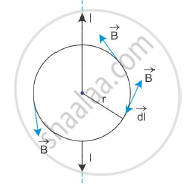# Find Magnetic Field Due to Straight Infinite Current Carrying Wire. - Physics

Use this law to find magnetic field due to straight infinite current carrying wire.

#### Solution

Magnetic field due to straight infinite current carrying wire:

The figure below shows a straight infinite current carrying wire around which a circular loop of radius r is shownThe field lines are circular, and hence, the field B at any point of the circular loop is directed along the tangent to the circle at that point. The magnitude of field B is the same at every point of the circular loop.

Therefore, we get

∫B.dl=∫Bdl cosθ = ∫Bdl cos0 = B∫dl =B(2πr)

Now, from Ampere’s circuital law, the above equation is

B(2pir)=mu_0I

:.B=(mu_0I)/(2pir)

Concept: Magnetic Force
Is there an error in this question or solution?
2015-2016 (March) All India Set 3 N

Share